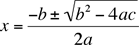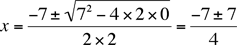Please show work and indicate "answer", using the quadratic formula - (x+2) (2x+3) = 6 I am tutoring my grandson in High School level Algebra II and it has been a number of years since I've done the quadratic formula, please help. thank you. Miriam Miriam, The quadratic formula says that if you have the quadratic equation a x2 + b x + c = 0 then you can find x byFor the problem that you sent the first task is to convert the equation into the form a x2 + b x + c = 0 Your equation is (x+2) (2x+3) = 6 First expand the left side to get 2 x2 + 7 x + 6 = 6 and the add -6 to both sides to make the right side zero. 2 x2 + 7 x = 0 This is now of the correct form a x2 + b x + c = 0 with a = 2, b = 7 and c = 0. Thus the general quadratic expression givesand thus x = 0 or x = -14/4 = -7/2  However, no one would ever use the general quadratic expression to solve 2 x2 + 7 x = 0 This expression factors to yield 2 x2 + 7 x = x(2x + 7) = 0 and hence x = 0 or x = -7/2  Penny